# PSEB 6th Class Maths MCQ Chapter 5 Fractions

Punjab State Board PSEB 6th Class Maths Book Solutions Chapter 5 Fractions MCQ Questions with Answers.

## PSEB 6th Class Maths Chapter 5 Fractions MCQ Questions

Multiple Choice Questions

Question 1.
Which of the following does not represent any fraction?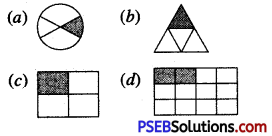Question 2.
Which of the following is a proper fraction?
(a) $$\frac {5}{5}$$
(b) $$\frac {12}{11}$$
(c) $$\frac {7}{9}$$
(d) 7
(c) $$\frac {7}{9}$$

Question 3.
Which of the following is an improper fraction?
(a) $$\frac {5}{8}$$
(b) $$2\frac {3}{4}$$
(c) $$\frac {7}{11}$$
(d) $$\frac {15}{16 }$$
(b) $$2\frac {3}{4}$$

Question 4.
The fractions having las numerator are called …………. fractions.
(a) Like
(b) Unlike
(c) Unit
(d) Proper.
(c) Unit

Question 5.
The fractions having same denominators are called …………. fractions.
(a) Proper
(b) Unit
(c) Improper
(d) Like.
(d) Like.

Question 6.
The fraction having different denominators are called ……………. fractions.
(a) Unlike
(b) Like
(c) Improper
(d) Unit.
(a) UnlikeQuestion 7.
Express 8 hours as a fraction of 1 day.
(a) $$\frac {2}{3}$$
(b) $$\frac {1}{3}$$
(c) $$\frac {8}{1}$$
(d) $$\frac {1}{8}$$
(b) $$\frac {1}{3}$$

Question 8.
Find : $$\frac {2}{5}$$ of ₹ 20.
(a) ₹ 8
(b) ₹ 10
(c) ₹ 12
(d) ₹ 40.
(a) ₹ 8

Question 9.
Write $$\frac {19}{4}$$ as mixed fraction.
(a) $$3\frac {4}{5}$$
(b) $$4\frac {4}{3}$$
(c) $$4\frac {3}{4}$$
(d) $$5\frac {1}{4}$$
(c) $$4\frac {3}{4}$$

Question 10.
$$7\frac {2}{3}$$ = ……………….
(a) $$\frac {17}{3}$$
(b) $$\frac {23}{3}$$
(c) $$\frac {13}{3}$$
(d) $$\frac {42}{3}$$
(b) $$\frac {23}{3}$$Question 11.
Which of the following represents an improper fraction?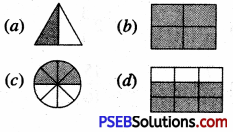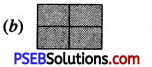Question 12.
Which of the following is an equivalent of $$\frac {5}{7}$$?
(a) $$\frac {25}{49}$$
(b) $$\frac {20}{35}$$
(c) $$\frac {35}{49}$$
(d) $$\frac {35}{28}$$
(c) $$\frac {35}{49}$$

Question 13.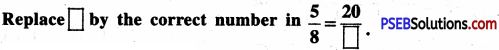(a) 32
(b) 24
(c) 40
(d) 16
(a) 32

Question 14.
Which of the following are in ascending order?
(a) $$\frac{2}{3}, \frac{2}{7}, \frac{2}{5}$$
(b) $$\frac{2}{3}, \frac{2}{5}, \frac{2}{7}$$
(c) $$\frac{2}{7}, \frac{2}{3}, \frac{2}{5}$$
(d) $$\frac{2}{7}, \frac{2}{5}, \frac{2}{3}$$
(d) $$\frac{2}{7}, \frac{2}{5}, \frac{2}{3}$$Question 15.
Which of the following are in descending order?
(a) $$\frac{1}{8}, \frac{1}{3}, \frac{1}{9}$$
(b) $$\frac{1}{3}, \frac{1}{8}, \frac{1}{9}$$
(c) $$\frac{1}{8}, \frac{1}{9}, \frac{1}{3}$$
(d) $$\frac{1}{3}, \frac{1}{9}, \frac{1}{8}$$
(b) $$\frac{1}{3}, \frac{1}{8}, \frac{1}{9}$$

Question 16.
$$\frac{4}{6}+\frac{3}{6}$$ = …………. .
(a) $$\frac {7}{12}$$
(b) $$\frac {7}{8}$$
(c) $$1\frac {1}{6}$$
(d) $$1\frac {1}{12}$$
(c) $$1\frac {1}{6}$$

Question 17.
$$\frac{4}{9}+\frac{5}{9}-\frac{2}{9}$$ = ……………. .
(a) $$\frac {7}{9}$$
(b) $$\frac {7}{18}$$
(c) $$\frac {11}{9}$$
(d) $$\frac {5}{9}$$
(a) $$\frac {7}{9}$$

Question 18.
$$\frac{2}{3}+\frac{1}{6}$$ = ………………
(a) $$\frac {3}{9}$$
(b) $$\frac {5}{6}$$
(c) $$\frac {7}{6}$$
(d) $$\frac {5}{9}$$
(b) $$\frac {5}{6}$$Question 19.
4 – $$\frac {1}{3}$$ = …………….
(a) $$4\frac {1}{3}$$
(b) $$3\frac {1}{3}$$
(c) $$4\frac {2}{3}$$
(d) $$3\frac {2}{3}$$
(d) $$3\frac {2}{3}$$

Question 20.
Divide $$\frac {1}{6}$$ by 2
(a) $$\frac {1}{3}$$
(b) $$\frac {1}{12}$$
(c) $$\frac {1}{18}$$
(d) 12
(b) $$\frac {1}{12}$$

Question 21.
Which fraction is represented by point P on the adjoining number line?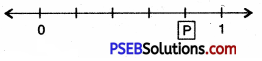(a) $$\frac {4}{5}$$
(b) $$\frac {5}{6}$$
(c) $$\frac {3}{4}$$
(d) $$\frac {3}{5}$$
(a) $$\frac {4}{5}$$

Question 22.
Which fraction is represented by point P on the adjoining number line?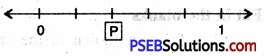(a) $$\frac {1}{2}$$
(b) $$\frac {3}{5}$$
(c) $$\frac {4}{5}$$
(d) $$\frac {2}{5}$$
(d) $$\frac {2}{5}$$Question 23.
Which of the following fractions are in ascending order?
(a) $$\frac{1}{2}, \frac{1}{3}, \frac{1}{4}$$
(b) $$\frac{5}{10}, \frac{5}{11}, \frac{5}{12}$$
(c) $$\frac{1}{10}, \frac{1}{100}, \frac{1}{1000}$$
(d) $$\frac{3}{10}, \frac{4}{10}, \frac{7}{10}$$
(d) $$\frac{3}{10}, \frac{4}{10}, \frac{7}{10}$$

Question 24.
Which of the following fractions are in descending order?
(a) $$\frac{1}{3}, \frac{1}{4}, \frac{1}{5}$$
(b) $$\frac{4}{11}, \frac{4}{12}, \frac{4}{13}$$
(c) $$\frac{1}{10}, \frac{1}{100}, \frac{1}{1000}$$
(d) $$\frac{7}{10}, \frac{9}{10}, \frac{11}{10}$$
(a) $$\frac{1}{3}, \frac{1}{4}, \frac{1}{5}$$

Question 25.
Which of the following fraction is shown in the shaded portion of the figure?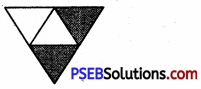(a) $$\frac {1}{3}$$
(b) $$\frac {2}{4}$$
(c) $$\frac {3}{4}$$
(d) $$\frac {2}{5}$$
(b) $$\frac {2}{4}$$

Fill in the blanks:

Question (i)
The fraction shown in the shaded portion of the figure is ……………..,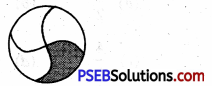$$\frac {1}{4}$$Question (ii)
The fraction of the shaded portion shown by the following figure is …………. .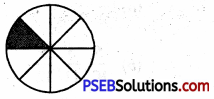$$\frac {1}{8}$$

Question (iii)
The fraction of the shaded portion shown by the given figure is …………… .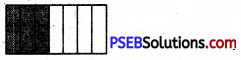$$\frac {3}{7}$$

Question (iv)
The upper part of the fraction is called ……………. .
numerator

Question (v)
The lower part of the fraction is called ……………. .
denominator

Write True/False:

Question (i)
The fraction $$\frac {3}{5}$$ is read as three fifth. (True/False)
TrueQuestion (ii)
The fraction whose numerator is less than the denominator is called the proper fraction. (True/False)
True

Question (iii)
$$\frac {7}{8}$$ is an improper fraction. (True/False)